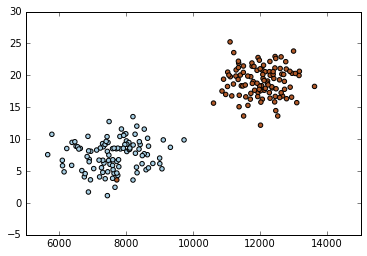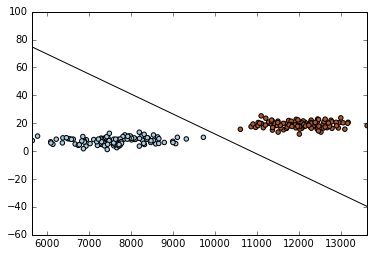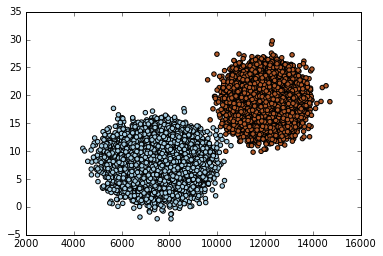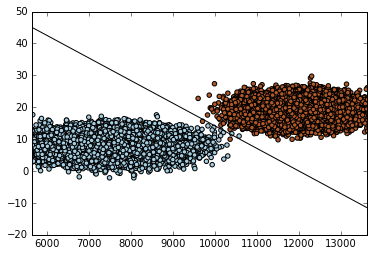• No products in the cart.

# 204.6.4 Building SVM model in Python

##### Building a SVM model in python.

Link to the previous post : https://statinfer.com/204-6-3-svm-the-algorithm/

We discussed the SVM algorithm in our last post. In this post we will try to build a SVM classification model in Python.

## SVM on Python

• There are multiple SVM libraries available in Python.
• The package ‘Scikit’ is the most widely used for machine learning.
• There is a function called svm() within ‘Scikit’ package.
• There are various options within svm() function to customize the training process.

### Practice : First SVM Learning Problem

• Dataset: Fraud Transaction/Transactions_sample.csv
• Draw a classification graph that shows all the classes
• Build a SVM classifier
• Draw the classifier on the data plots
• Predict the (Fraud vs not-Fraud) class for the data points Total_Amount=11000, Tr_Count_week=15 & Total_Amount=2000, Tr_Count_week=4
• Draw a classification graph that shows all the classes
• Build a SVM classifier
• Draw the classifier on the data plots
In :
```# Importing the sample data
import pandas as pd
X = Transactions_sample[['Total_Amount']+['Tr_Count_week']]  # we only take the first two features. We could
# avoid this ugly slicing by using a two-dim dataset
y = Transactions_sample[['Fraud_id']].values.ravel()
```
In :
```#Drawing a classification graph of all classes
import matplotlib.pyplot as plt

plt.scatter(X['Total_Amount'], X['Tr_Count_week'], c=y, cmap=plt.cm.Paired)
plt.show()
```In :
```#Building a SVM Classifier in python
from sklearn import svm
import numpy
X = Transactions_sample[['Total_Amount']+['Tr_Count_week']]  # we only take the first two features. We could
# avoid this ugly slicing by using a two-dim dataset
y = Transactions_sample[['Fraud_id']].values.ravel()

clf = svm.SVC(kernel='linear')
model =clf.fit(X,y)

Predicted = numpy.zeros(50)

# NOTE: If i is in range(0,n), then i takes vales [0,n-1]
for i in range(0,50):
a = Transactions_sample.Total_Amount[i]
b = Transactions_sample.Tr_Count_week[i]
Predicted[i]=clf.predict([[a,b]])
del a,b
```
In :
```#Plotting in SVM
import matplotlib.pyplot as plt
plt.scatter(X['Total_Amount'], X['Tr_Count_week'], c=y, cmap=plt.cm.Paired)
w = clf.coef_
o = -w / w
plt.xlim(min(Transactions_sample.Total_Amount), max(Transactions_sample.Total_Amount))
x_min, x_max = ax1.get_xlim()
xx = np.linspace(x_min, x_max)
yy = o * xx - (clf.intercept_) / w

plt.plot(xx, yy, 'k-')
plt.show()
```In :
```#Predict the (Fraud vs not-Fraud) class for the data points Total_Amount=11000, Tr_Count_week=15 & Total_Amount=2000, Tr_Count_week=4
#Prediction in SVM
new_data1=[11000, 15]
new_data2=[2000,4]

#Predict the (Fraud vs not-Fraud) class for the data points Total_Amount=11000, Tr_Count_week=15 & Total_Amount=2000, Tr_Count_week=4
NewPredicted1=model.predict([new_data1])
print(NewPredicted1)

NewPredicted2=clf.predict([new_data2])
print(NewPredicted2)
```
```

```
In :
```# Importing the whole dataset
import pandas as pd
X = Transactions[['Total_Amount']+['Tr_Count_week']]  # we only take the first two features. We could
# avoid this ugly slicing by using a two-dim dataset
y = Transactions[['Fraud_id']].values.ravel()
```
In :
```#Drawing a classification graph of all classes
import matplotlib.pyplot as plt

plt.scatter(X['Total_Amount'], X['Tr_Count_week'], c=y, cmap=plt.cm.Paired)
plt.show()
```In :
```#Build a SVM classifier
clf = svm.SVC(kernel='linear')
Smodel =clf.fit(X,y)
```
In :
```#Plotting in SVM
import matplotlib.pyplot as plt
plt.scatter(X['Total_Amount'], X['Tr_Count_week'], c=y, cmap=plt.cm.Paired)
w = clf.coef_
o = -w / w
plt.xlim(min(Transactions_sample.Total_Amount), max(Transactions_sample.Total_Amount))
x_min, x_max = ax1.get_xlim()
xx = np.linspace(x_min, x_max)
yy = o * xx - (clf.intercept_) / w
plt.plot(xx, yy, 'k-')
plt.show()
```The next post is about the non-linear decision boundary.
Link to the next post : https://statinfer.com/204-6-5-the-non-linear-decision-boundary/
21st June 2017
0 responses on "204.6.4 Building SVM model in Python"